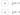A key component of quantum computing is the Hadamard gate. Used in many different kinds of quantum algorithms, it is often the first gate presented to students. To define the Hadamard gate, which we denote with the letter H, we need the following matrix:

Using the formula [1/sqrt(2) 1/sqrt(2)], To simplify, write: [1/sqrt(2) -1/sqrt(2)]

When applied to a qubit, the Hadamard gate causes it to simultaneously occupy both the |0| and |1| states. When a qubit is in the |0 state before passing through the Hadamard gate, it transitions to the |1 state.

One plus one square root two is equal to two zeros and one.

Similarly, if the qubit is in the |1 state to begin with, the Hadamard gate will change it to the state:

(0 - (1/sqrt(2))|0) + (1/sqrt(2))|1)

To put it another way, the Hadamard gate spins the qubit such that it is centred halfway around the Bloch sphere. A common metaphor for this is a 90-degree rotation of the state vector around the Y-axis of the Bloch sphere.

The fact that the Hadamard gate is also its own inverse is one of its most distinctive features. To rephrase, applying the Hadamard gate to a qubit twice in a row restores it to its initial state. Because of this, the Hadamard gate may be used to create and destroy superpositions.

In addition to its application in the Deutsch-Jozsa algorithm and the quantum Fourier transform, the Hadamard gate is a key component in a wide variety of other quantum algorithms. The Deutsch-Jozsa technique may check whether a function is constant or balanced with a single query by using the Hadamard gate to generate a superposition of all potential input states. An input state is converted into a frequency-space representation using the quantum Fourier transform, which uses the Hadamard gate to generate a superposition of all possible output states.

The Hadamard gate is essential for generating entangled states and is therefore very useful in quantum algorithms. For instance, the Bell state is produced when two qubits in the |0 state are subjected to a Hadamard gate and subsequently subjected to a CNOT gate between them.

(one square root of two)(00) plus (one square root of two)(11)

In quantum communication and error correction, this condition of maximum entanglement is very useful.

In conclusion, the Hadamard gate is a basic gate in quantum computing that is used to construct and undo superpositions, translate states into frequency space representations, and generate entangled states. Because of its superposition capabilities and straightforward matrix structure, it may be used in a wide variety of quantum computing applications.

XPERIMEN.COM